×#### Thank you for registering.

One of our academic counsellors will contact you within 1 working day.

Click to Chat

1800-1023-196

+91-120-4616500

CART 0

• 0

MY CART (5)

Use Coupon: CART20 and get 20% off on all online Study Material

ITEM
DETAILS
MRP
DISCOUNT
FINAL PRICE
Total Price: Rs.

There are no items in this cart.
Continue Shopping• Complete JEE Main/Advanced Course and Test Series
• OFFERED PRICE: Rs. 15,900
• View Details

```Chapter 9: Arithmetic Progressions Exercise – 9.1

Question: 1

Write the first terms of each of the following sequences whose nth term are

(i) an = 3n + 2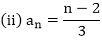(iii) an = 3n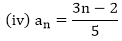(v) an = (-1)n2n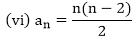(vii) an = n2 - n + 1

(viii) an = n2 - n + 1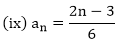Solution:

We have to write first five terms of given sequences

(i) an = 3n + 2

Given sequence an = 3n + 2

To write first five terms of given sequence put n = 1, 2, 3, 4, 5, we get

a1 = (3 × 1) + 2 = 3 + 2 = 5

a2 = (3 × 2) + 2 = 6 + 2 = 8

a3 = (3 × 3) + 2 = 9 + 2 = 11

a4 = (3 × 4) + 2 = 12 + 2 = 14

a5 = (3 × 5) + 2 = 15 + 2 = 17

∴ The required first five terms of given sequence an = 3n + 2 are 5, 8, 11, 14, 17.Given sequence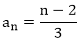Put n = 1, 2, 3, 4, 5 then we get∴ The required first five terms of given sequence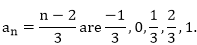(iii) an = 3n

Given sequence an = 3n

To write first five terms of given sequence, put n = 1, 2, 3, 4, 5 in given sequence then,

a1 = 31 = 3; a2 = 32 = 9; a3 = 27; a4 = 34 = 81; a5 = 35 = 243.Given sequence,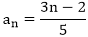To write first five terms, put n = 1, 2, 3, 4, 5 in given sequenceThen, we get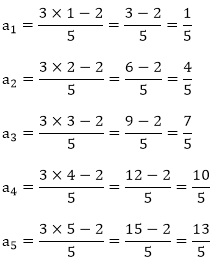∴ The required first five terms are 1/5, 4/5, 7/5, 10/5, 13/5

(v) an = (-1)n2n

Given sequence is an = (-1)n2n

To get first five terms of given sequence an, put n = 1, 2, 3, 4, 5.

a1 = (-1)1.21 = (-1).2 = -2

a2 = (-1)2.22 = (-1).4 = -4

a3 = (-1)3.23 = (-1).8 = -8

a4 = (-1)4.24 = (-1).16 = 16

a5 = (-1)5.25 = (-1).32 = -32

∴ The first five terms are – 2, 4, - 8, 16, - 32.The given sequence is,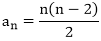To write first five terms of given sequencePut n = 1, 2, 3, 4, 5. Then, we get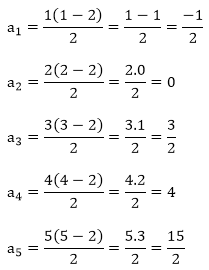∴  The required first five terms are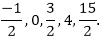(vii) an = n2 - n + 1

The given sequence is, an = n2 - n + 1

To write first five terms of given sequence an1 we get put n = 1, 2, 3, 4, 5. Then we get

a1 = 12 - 1 + 1 = 1

a2 = 22 - 2 + 1 = 3

a3 = 32 - 3 + 1 = 7

a4 = 42 - 4 + 1 = 13

a5 = 52 - 5 + 1 = 21

∴ The required first five terms of given sequence an = n2 - n + 1 are 1, 3, 7, 13, 21

(viii) an = 2n2 - 3n + 1

The given sequence is an = 2n2 - 3n + 1

To write first five terms of given sequence and, we put n = 1, 2, 3, 4, 5. Then we get

a1 = 2.12 - 3.1 + 1 = 2 - 3 + 1 = 0

a2 = 2.22 - 3.2 + 1 = 8 - 6 + 1 = 3

a3 = 2.32 - 3.3 + 1 = 18 - 9 + 1 = 10

a4 = 2.42 - 3.4 + 1 = 32 - 12 + 1 = 21

a5 = 2.52 - 3.5 + 1 = 50 - 15 + 1 = 36

∴ The required first five terms of given sequence an - 2n2 - 3n + 1 are 0, 3, 10, 21, 36Given sequence is,To write first five terms of given sequence we put n = 1, 2, 3, 4, 5. Then, we get,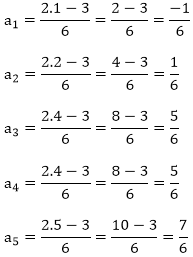∴ The required first five terms of given sequence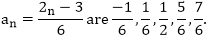Question: 2

Find the indicated terms in each of the following sequences whose nth terms are:

(i) an = 5n - 4; a12 and a15(iii) an = n(n - 1)(n - 2); a5 and a8

(iv) an = (n - 1)(2 - n)(3 + n);a11 a21 a3

(v) an = (-1)nn;a3, a5, a8

Solution:

We have to find the required term of a sequence when nth term of that sequence is given:

(i) an = 5n - 4; a12 and a15

Given nth term of a sequence an = 5n - 4

To find 12th terms, 15th terms of that sequence, we put n = 12 15 in its nth term.

Then, we get

a12 = 5.12 - 4 = 60 - 4 = 56

a15 = 5.15 - 4 = 15 - 4 = 71

∴ The required terms a12 = 56, a15 = 71Given nth term is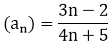To find 7th, 8th terms of given sequence, we put n = 7, 8.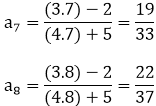∴ The required terms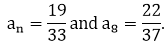(iii) an = n(n - 1)(n - 2); a5 and a8

Given nth term is an = n(n - 1)(n - 2)

To find 5th, 8th terms of given sequence, put n = 5, 8 in an then, we get

a5 = 5(5 - 1).(5 - 2) = 5.4.3 = 60

a8 = 8.(8 - 1).(8 - 2) = (8.7.6) = 336

∴ The required terms are a5 = 60 and a8 = 336

(iv) an = (n - 1)(2 - n)(3 + n);a11 a21 a3

The given nth term is an = (n + 1)(2 - n)(3 + n)

To find a1, a2, a3 of given sequence put n = 1, 2, 3 is an

a1 = (1 - 1)(2 - 1)(3 + 1) = 0.1.4 = 0

a2 = (2 - 1)(2 - 2)(3 + 2) = 1.0.5 = 0

a3 = (3 - 1)(2 - 3)(3 + 3) = 2.-1.6 = -12

∴ The required terms a1 = 0, a2 = 0, a3 = -12

(v) an = (-1)nn; a3, a5, a8

The given nth term is, an = (-1)n.n

To find a3, a5, a8 of given sequence put n = 3, 5, 8, in an.

a3 = (-1)3.3 = -1.3 = -3

a5 = (-1)5.5 = -1.5 = -5

a8 = (-1)8 = 1.8 = 8

∴ The required terms a3 = -3, a5 = -5, a8 = 8

Question: 3

Find the next five terms of each of the following sequence given by:

(i) a1 = 1, 1n = an-1 + 2, n ≥ 2

(ii) a1 = a2 = 2, an = an-1 - 3, n > 2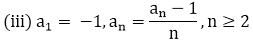(iv) a1 = 4, an = 4 an-1 + 3, n > 1

Solution:

We have to find next five terms of following sequences.

(i) a1 = 1, an = an-1 + 2, n ≥ 3

Given first term (a1) = 1,

nth term an = an-1 + 2, n ≥ 2

To find 2nd, 3rd, 4th, 5th, 6th terms, we use given condition n ≥ 2 for nth term an = an-1 + 2

a2 = a2-1 + 2 = a1 + 2 = 1 + 2 = 3 (∴ a1 = 1)

a3 = a3-1 + 2 = a2 + 2 = 3 + 2 = 5

a4 = a4-1 + 2 = a3 + 2 = 5 + 2 = 7

a5 = a5-1 + 2 = a4 + 2 = 7 + 2 = 9

a6 = a6-1 + 2 = a5 + 2 = a + 2 = 11

∴ The next five terms are,

a2 = 3, a3 = 5, a4 = 7, a5 = a, a6 = 11

(ii) a1 = a2 = 2, an = an-1 - 3, n > 2

Given,

First term (a1) = 2

Second term (a2) = 2

nth term (an) = an-1 - 3

To find next five terms i.e., a3, a4, a5, a6, a7 we put n = 3, 4, 5, 6, 7 is an

a3 = a3-1 - 3 = 2 - 3 = – 1

a4 = a4-1 - 3 = a3 - 3 = – 1 - 3 = – 4

a5 = a5-1 - 3 = a4 - 3 = -4 - 3 = -7

a6 = a6-1 - 3 = a5 - 3 = -7 - 3 = -10

a7 = a7-1 - 3 = a6 - 3 = -10 - 3 = -13

∴ The next five terms are, a3 = -1, a4 = – 4, a5 = -7, a6 = -10, a7 = -13Given, first term (a1) = – 1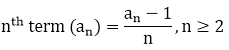To find next five terms i.e., a2, a3, a4, a5, a6 we put n = 2, 3, 4, 5, 6 is an∴ The next five terms are,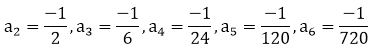(iv) a1 = 4, an = 4 an-1 + 3, n > 1

Given,

First term (a1) = 4

nth term (an) = 4 an-1 + 3, n > 1

To find next five terms i.e., a2, a3, a4, a5, a6 we put n = 2, 3, 4, 5, 6 is an

Then, we get

a2 = 4a2-1 + 3 = 4. a1 + 3 = 4.4 + 3 = 19 (∴ a1 = 4)

a3 = 4a3-1 + 3 = 4.a2 + 3 = 4(19) + 3 = 79

a4 = 4a4-1 + 3 = 4.a3 + 3 = 4(79) + 3 = 319

a5 = 4a5-1 + 3 = 4.a4 + 3 = 4(319) + 3 = 1279

a6 = 4a6-1 + 3 = 4.a5 + 3 = 4(1279) + 3 = 5119

∴ The required next five terms are,

a2 = 19, a3 = 79, a4 = 319, a5 = 1279, a6 = 5119
```### Course Features

• 728 Video Lectures
• Revision Notes
• Previous Year Papers
• Mind Map
• Study Planner
• NCERT Solutions
• Discussion Forum
• Test paper with Video Solution